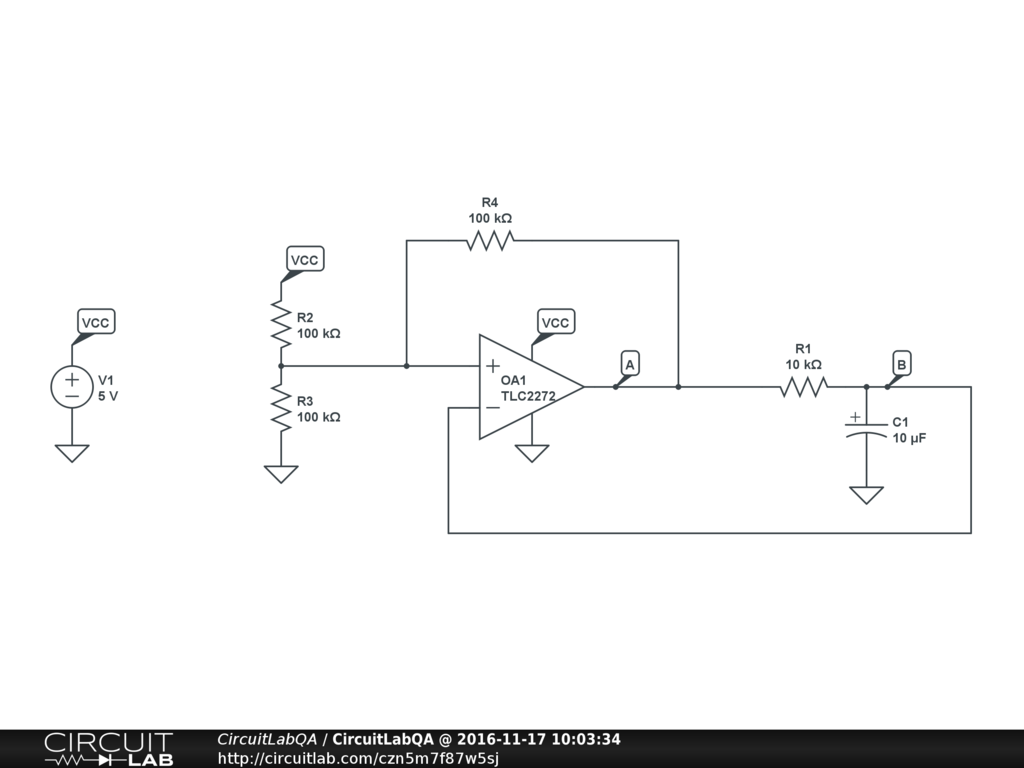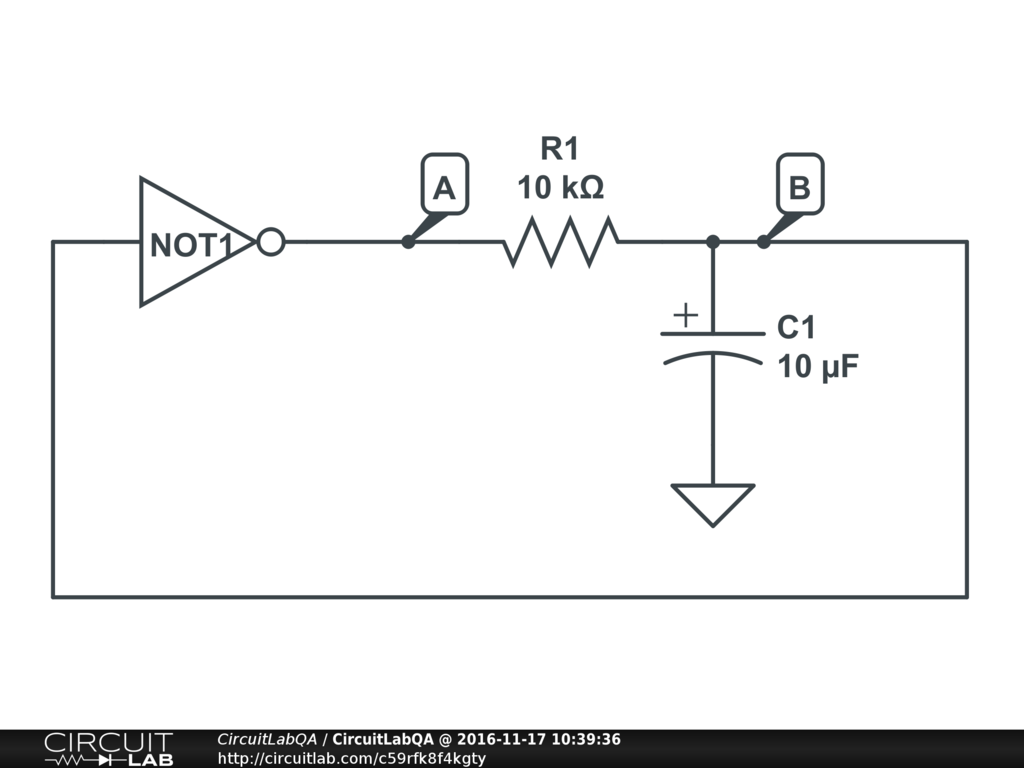## Precision capacitor measurement with op amp, resistors, oscilloscope? SOLVED

 Using only op amps, resistors, and a normal lab oscilloscope, how can you make a precise measurement of the capacitance of an unknown capacitor? This is a bonus question on my problem set but it sounds crazy. by nitabobby64 November 17, 2016

 One way would be to make an oscillator using (known) resistances and the (unknown) capacitor. This uses the op-amp more as a comparator than an op-amp, but I think it should count... Take a look at this simulation:Open and run it! C1 is your unknown capacitor, and $\tau = R_1 C_1$ is the RC time constant. If you don't even know what order of magnitude C1 is, you can try a bunch of different R1 values so you get the time period into a place you can measure it accurately enough. Basically, R2, R3, and R4 form a voltage divider. When $V_A = V_{CC}$ then the non-inverting input of the op amp is at $\frac {2} {3} V_{CC} = 3.33$ volts. And when $V_A = 0$ then the non-inverting input is at $\frac {1} {3} V_{CC} = 1.67$ volts. Pretend that when the circuit starts, the capacitor is discharged, so $V_B=0$ and therefore since the non-inverting input is higher, the op-amp output $V_A=5$. This will start charging the capacitor through R1, and it will keep charging exponentially until it crosses the non-inverting input at 3.33 volts. At that time, the op-amp inverting input will be higher than the non-inverting input, so the output will swing the other way to $V_A=0$. At that point the capacitor will start discharging, again until it hits 1.67 volts... and at that time it switches again and the cycle starts over! Tada -- oscillator! How long does it take to switch? Well, how long does it take to charge an RC-combination from 1.67 up to 3.33 volts (or from 1/3rd to 2/3rds of full voltage)? If the charging-up voltage as a function of time looks like $$V_B(t) = 5 (1 - e^{-\frac {t} {\tau}})$$, then we can figure out for $V_B(t_1) = 5 * \frac {1} {3}$ and $V_B(t_1) = 5 * \frac {2} {3}$... that's $$\frac {1} {3} = 1 - e^{-\frac {t_1} {\tau}}$$ $$\frac {2} {3} = e^{-\frac {t_1} {\tau}}$$ $$ln(\frac {2} {3}) = -\frac {t_1} {\tau}$$ $$ln(\frac {2} {3}) = -\frac {t_1} {\tau}$$ $$t_1 = \tau * ln(\frac {3} {2})$$ You can do the same thing for $t_2$ and you'll get $$t_2 = \tau * ln(3)$$ The difference is $$t_2 - t_1 = \tau * \big( ln(3) - ln(\frac {3} {2}) \big)$$ $$t_2 - t_1 = \tau * ln(2)$$ That's our half-period -- the time to go from 1/3rd to 2/3rds VCC. But we have to discharge too to complete the cycle, so I'll call our overall oscillator period $T$. Well, $$T= 2 * (t_2 - t_1)$$ $$T= 2 ln(2) * R_1 C_1$$ If you plug in our values in the schematic above, you get $T =139$ milliseconds. Does that match the simulation? You bet it does :) So if the capacitance was unknown you could just measure the period and reverse the formula: $$C_1 = \frac {T} {2 ln(2) * R_1}$$ ACCEPTED +2 votes by mrobbins November 17, 2016

 @mrobbins perfect. Just wanted to point out that you could also do this with a digital comparator chip (it would look the same as your circuit). Or if you had an inverter like a 7404 laying around, its even simpler...Simulation works too! Same basic idea. The oscillation period $T$ will be different than the op-amp one because it the inverter will have different thresholds (not exactly 1/3rds or 2/3rds of $V_{CC}$), but if you have an oscilloscope you can measure those too and fix your math. +1 vote by berniekorrie November 17, 2016

CircuitLab's Q&A site is a FREE questions and answers forum for electronics and electrical engineering students, hobbyists, and professionals.

We encourage you to use our built-in schematic & simulation software to add more detail to your questions and answers.

Acceptable Questions:

• Concept or theory questions
• Practical engineering questions
• “Homework” questions
• Software/hardware intersection
• Best practices
• Design choices & component selection
• Troubleshooting

Unacceptable Questions:

• Non-English language content
• Non-question discussion
• Non-electronics questions
• Vendor-specific topics
• Pure software questions
• CircuitLab software support

Please respect that there are both seasoned experts and total newbies here: please be nice, be constructive, and be specific!